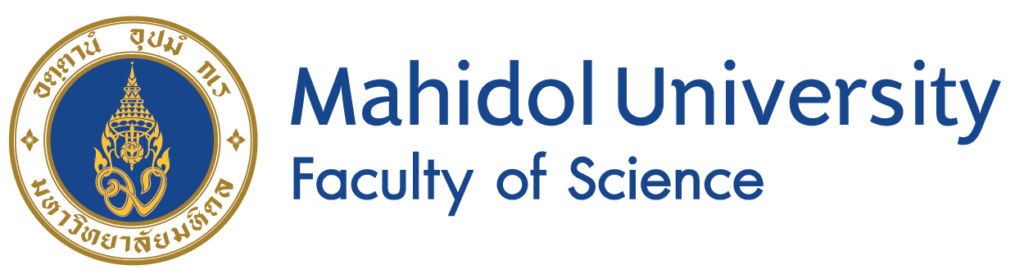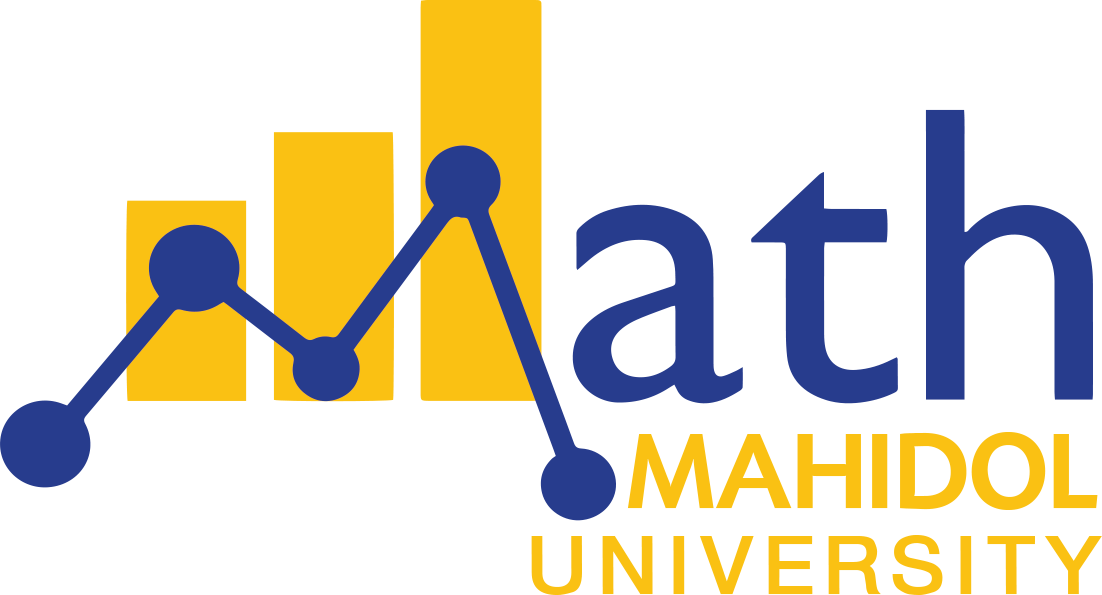Search

## Theoretical Mathematics & Analysis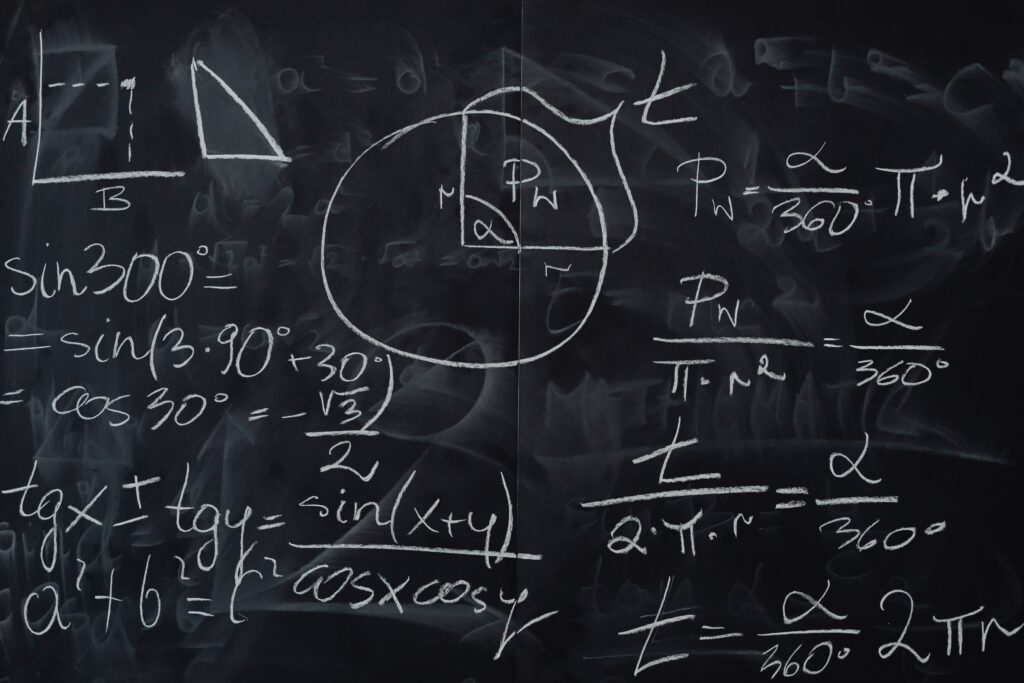Research studies in theoretical mathematics, or pure mathematics, are generally concerned with understanding various structures that appear in mathematics and developing mathematical theory behind them. Results in theoretical mathematics become foundation to other areas such as applied mathematics and statistics as well as computer science and physics.

### Research themes

Analysis is the branch of mathematics studying continuity, differentiation, and integration, which can be seen as continuation of calculus. The main objects of interest in research are real-valued functions, complex-valued functions, spaces of functions, and measures. Understanding analysis of functions and differential equations are important in numerical methods and mathematical modeling used frequently in the Applied Mathematics track.

Geometry and topology is the branch of mathematics studying measurement and shape of objects. Research in geometry goes beyond Euclidean geometry by looking at more general metric spaces or studying manifolds, which are higher dimensional analogue of curves and surfaces. Topology is a study of shapes or properties that are not changed under deformation. Shape of our universe and knots in our cells are examples of geometric objects appearing in other disciplines. Techniques from geometry and topology are sometimes used in visualizing big data studied in the Industrial Mathematics and Data Science program.

Discrete mathematics is the branch of mathematics studying objects which are not continuous and often involves counting (Combinatorics). Graph theory is the area that focuses on graphs which usually come from studying networks. Graph theory has application to various fields such as physics, chemistry, biology, and social sciences. In particular, research in graph theory can be used to understand data structures, network communications and related algorithms appearing in the Computer Science track.

### Related programs

Members of the group involve in the research of the following programs:

• BSc Mathematics
• PhD Mathematics

They also teach fundamental courses on the following programs:

• BSc Actuarial Science
• BSc Industrial Mathematics & Data Science
• MSc Applied Mathematics

### Recent publications

On the geometry of Cayley automatic groups

Berdinsky D, Elder M, Taback J

International Journal of Algebra and Computation, 2022

Multidimensional Fibonacci coding

Pooksombat P, Udomkavanich P, Kositwattanarerk W

Mathematics, 2022

Superbridge and bridge indices for knots

Adams C, Agarwal N, Allen R, Khandhawit T, Simons A, Winarski R

Journal of Knot Theory and Its Ramifications, 2021

Being Cayley automatic is closed under taking wreath product with virtually cyclic groups

Berdinsky D, Elder M, Taback J

Bulletin of the Australian Mathematical Society, 2021

The solutions of some Riemann–Liouville fractional integral equations

Kaewnimit K, Wannalookkhee F, Nonlaopon K, Orankitjaroen S.

Fractal and Fractional, 2021

### People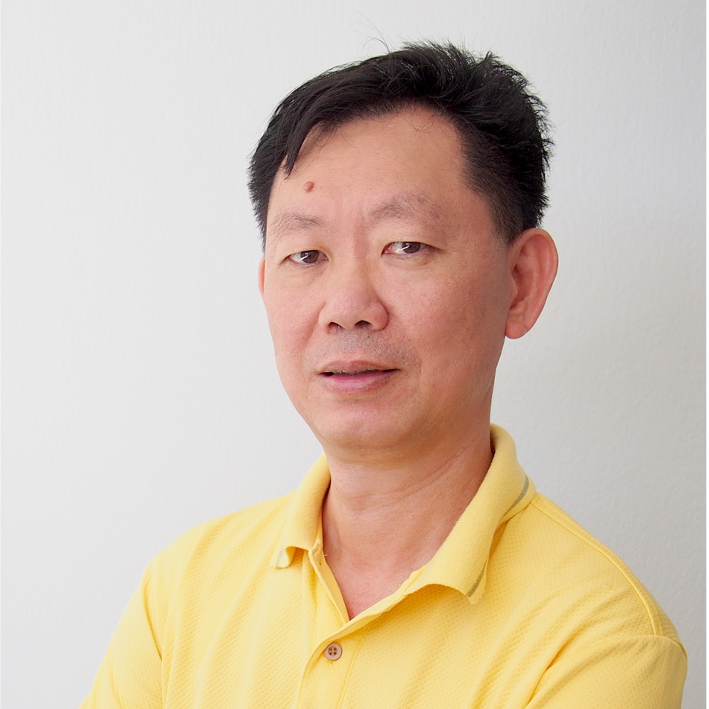Chaiwat Maneesawarng
Assistant Professor
( Theoretical Mathematics)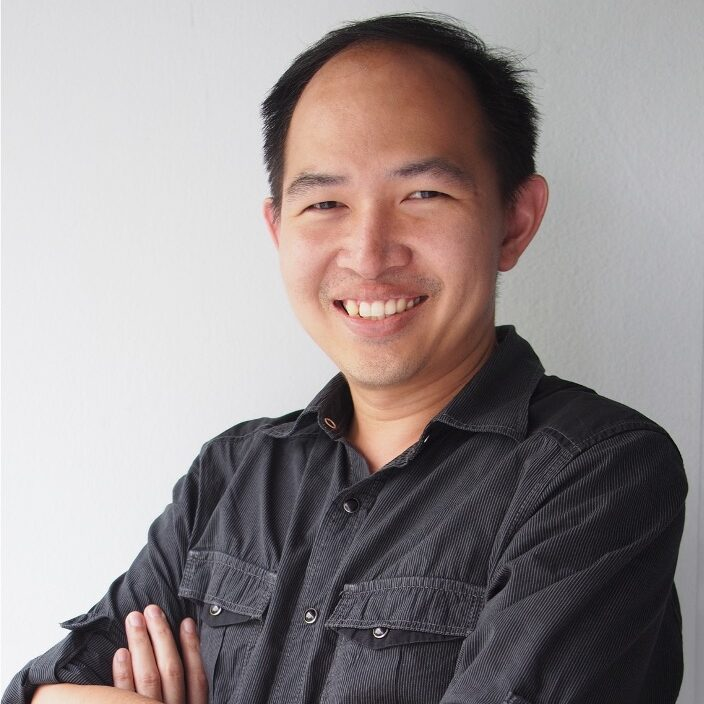Chanun Lewchalermvongs
Assistant Professor
( Theoretical Mathematics)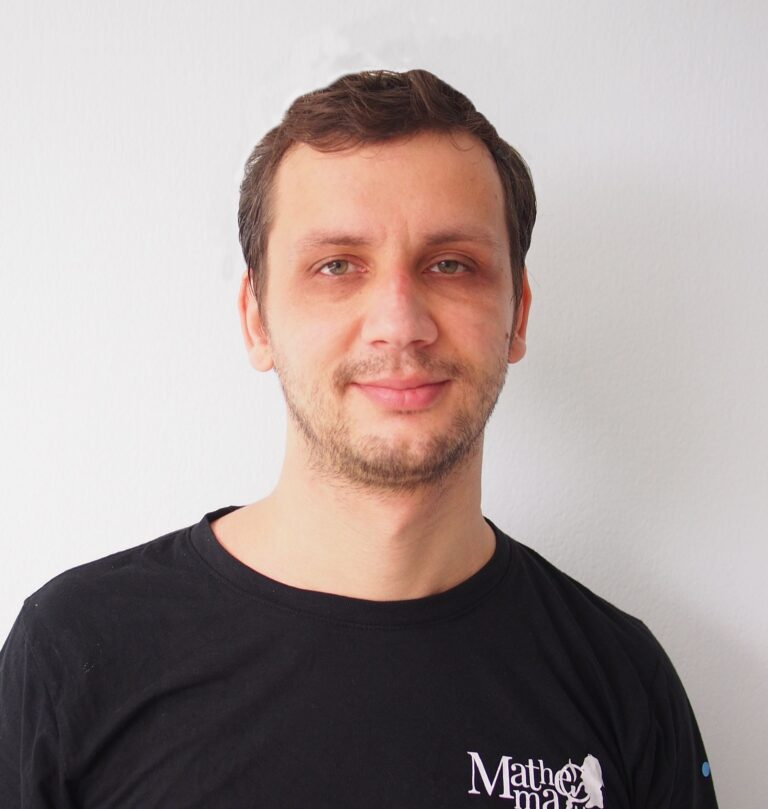Dmitry Berdinsky
Lecturer
(Theoretical Mathematics)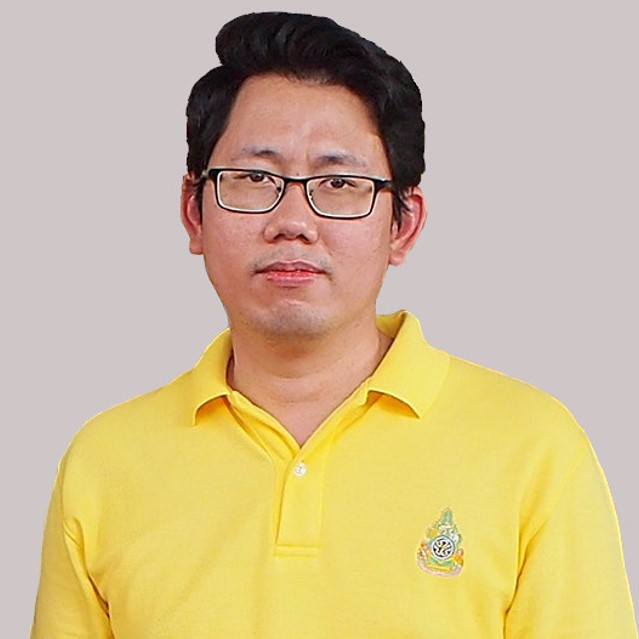Nattapong Bosuwan
Associate Professor
(Theoretical Mathematics)Nguyen Van Sanh
Lecturer
(Theoretical Mathematics)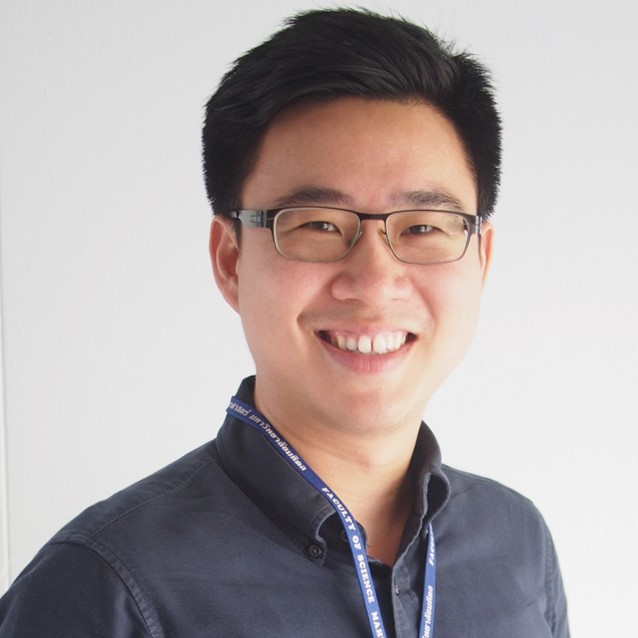Pichkitti Bannangkoon
Lecturer
(Theoretical Mathematics)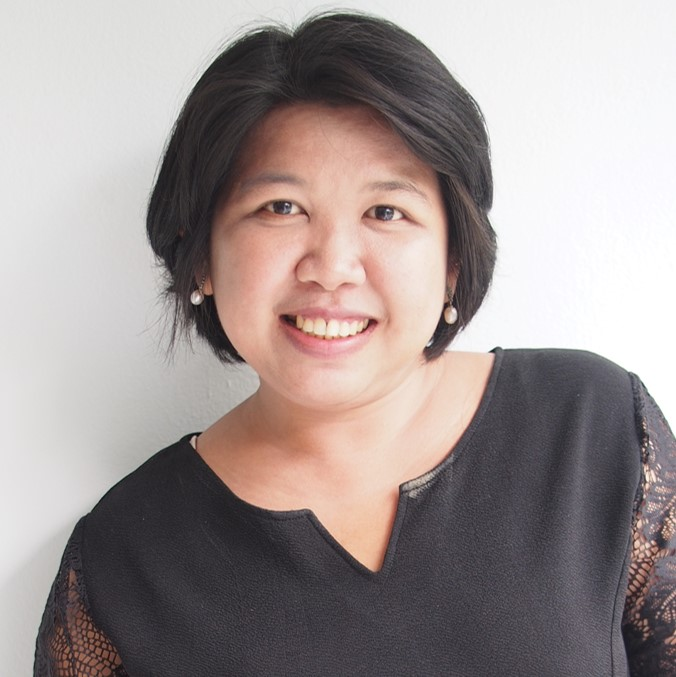Piyanan Pasom
Lecturer
(Theoretical Mathematics)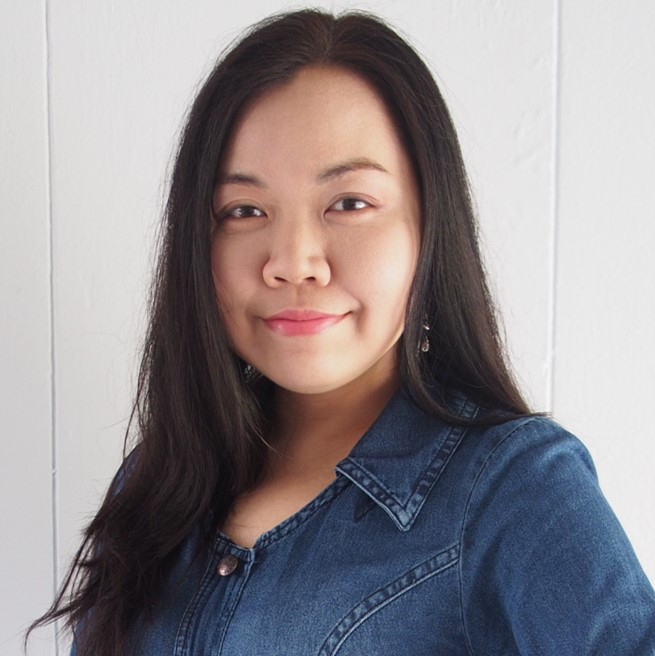Ruth J. Skulkhu
Assistant Professor
(Theoretical Mathematics)Somsak Orankitjaroen
Assistant Professor
(Theoretical Mathematics)Tirasan Khandhawit
Lecturer
(Theoretical Mathematics)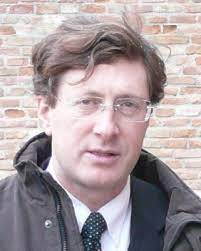Andrea De Gaetano